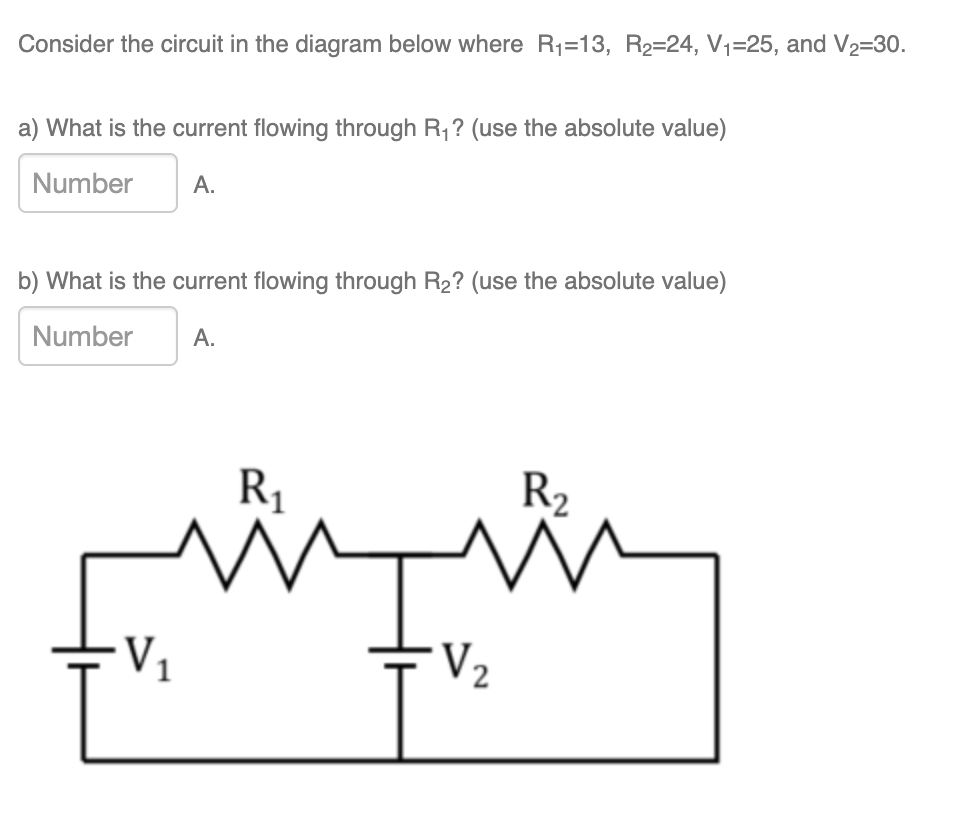# Question Consider the circuit in the diagram below where Ri=13, R2=24, V1=25, and V2=30. a) What is the current flowing through Ry? (use the absolute value) Number A. b) What is the current flowing through R2? (use the absolute value) Number A. R R2 T. M V V2